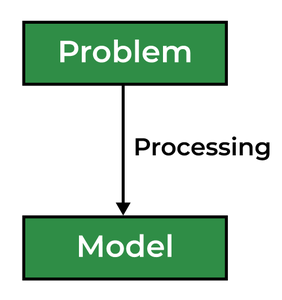GFG App
Open AppBrowser
Continue

# Abstraction in C++

Data abstraction is one of the most essential and important features of object-oriented programming in C++. Abstraction means displaying only essential information and hiding the details. Data abstraction refers to providing only essential information about the data to the outside world, hiding the background details or implementation.

Consider a real-life example of a man driving a car. The man only knows that pressing the accelerator will increase the speed of the car or applying brakes will stop the car but he does not know how on pressing the accelerator the speed is actually increasing, he does not know about the inner mechanism of the car or the implementation of the accelerator, brakes, etc in the car. This is what abstraction is.

## Types of Abstraction:

1. Data abstraction – This type only shows the required information about the data and hides the unnecessary data.
2. Control Abstraction – This type only shows the required information about the implementation and hides unnecessary information.## Abstraction using Classes

We can implement Abstraction in C++ using classes. The class helps us to group data members and member functions using available access specifiers. A Class can decide which data member will be visible to the outside world and which is not.

One more type of abstraction in C++ can be header files. For example, consider the pow() method present in math.h header file. Whenever we need to calculate the power of a number, we simply call the function pow() present in the math.h header file and pass the numbers as arguments without knowing the underlying algorithm according to which the function is actually calculating the power of numbers.

## Abstraction using Access Specifiers

Access specifiers are the main pillar of implementing abstraction in C++. We can use access specifiers to enforce restrictions on class members. For example:

• Members declared as public in a class can be accessed from anywhere in the program.
• Members declared as private in a class, can be accessed only from within the class. They are not allowed to be accessed from any part of the code outside the class.

We can easily implement abstraction using the above two features provided by access specifiers. Say, the members that define the internal implementation can be marked as private in a class. And the important information needed to be given to the outside world can be marked as public. And these public members can access the private members as they are inside the class.

Example

## C++

 `// C++ Program to Demonstrate the` `// working of Abstraction` `#include ` `using` `namespace` `std;`   `class` `implementAbstraction {` `private``:` `    ``int` `a, b;`   `public``:` `    ``// method to set values of` `    ``// private members` `    ``void` `set(``int` `x, ``int` `y)` `    ``{` `        ``a = x;` `        ``b = y;` `    ``}`   `    ``void` `display()` `    ``{` `        ``cout << ``"a = "` `<< a << endl;` `        ``cout << ``"b = "` `<< b << endl;` `    ``}` `};`   `int` `main()` `{` `    ``implementAbstraction obj;` `    ``obj.set(10, 20);` `    ``obj.display();` `    ``return` `0;` `}`

Output

```a = 10
b = 20```

You can see in the above program we are not allowed to access the variables a and b directly, however, one can call the function set() to set the values in a and b and the function display() to display the values of a and b.

• Helps the user to avoid writing the low-level code
• Avoids code duplication and increases reusability.
• Can change the internal implementation of the class independently without affecting the user.
• Helps to increase the security of an application or program as only important details are provided to the user.
• It reduces the complexity as well as the redundancy of the code, therefore increasing the readability.

## C++

 `#include` `using` `namespace` `std;`   `class` `Vehicle` `{` `    ``public``:` `        ``void` `company()` `        ``{` `            ``cout<<``"GFG\n"``;` `        ``}` `    ``public``:` `        ``void` `model()` `        ``{` `            ``cout<<``"SIMPLE\n"``;` `        ``}` `    ``public``:` `        ``void` `color()` `        ``{` `            ``cout<<``"Red/GREEN/Silver\n"``;` `        ``}` `    ``public``:` `        ``void` `cost()` `        ``{` `            ``cout<<``"Rs. 60000 to 900000\n"``;` `        ``}` `    ``public``:` `        ``void` `oil()` `        ``{` `            ``cout<<``"PETRO\n"``;` `        ``}` `    ``private``:` `        ``void` `piston()` `        ``{` `            ``cout<<``"4 piston\n"``;` `        ``}` `    ``private``:` `        ``void` `manWhoMade()` `        ``{` `            ``cout<<``"Markus Librette\n"``;` `        ``}` `};` `int` `main()` `{` `    `  `    ``Vehicle obj;` `    ``obj.company();` `    ``obj.model();` `    ``obj.color();` `    ``obj.cost();` `    ``obj.oil();` `}`

Output

```GFG
SIMPLE
Red/GREEN/Silver
Rs. 60000 to 900000
PETRO```

My Personal Notes arrow_drop_up